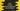# 3 different ways in Java to convert comma-separated strings to an ArrayList## How to convert comma-separated strings to an ArrayList in Java:

Suppose one string with comma-separated words is given and we need to convert it to an ArrayList in Java. We can do that in different ways.  In this post, we will learn different ways to convert a comma-separated string to an ArrayList in Java.

### Method 1: By using split:

We can use the split method to split all words in the string. This method takes one parameter and splits all words based on that.

For example, if our string is hello,world, we can pass a comma to the split method. This will return an array of strings. We can convert that array to a list and that list to an arraylist.

Below is the complete program:

``````import java.util.ArrayList;
import java.util.Arrays;

class Example{
public static void main(String[] args) {
String givenString = "one,two,three,four,five";

String[] splittedStrings = givenString.split(",");

ArrayList<String> arrayList = new ArrayList<>(Arrays.asList(splittedStrings));

System.out.println(arrayList);
}
}``````

Here,

• givenString is the string with words separated by comma.
• *splittedStrings *is created by calling split to givenString and we passed comma to it.
• arrayList is the final ArrayList created from this string array.
• The last line is printing the array list.

It will give one output as like below: ![Java program to convert comma separated strings to arraylist]](../images/java-comma-separated-strings-arraylist.png))

### Method 2: By using the stream API:

We can also the stream API to create a stream and collect it in a List.

``````import java.util.ArrayList;
import java.util.stream.Collectors;
import java.util.stream.Stream;

class Example{
public static void main(String[] args) {
String givenString = "one,two,three,four,five";

String[] splittedStrings = givenString.split(",");

ArrayList<String> arrayList = Stream.of(splittedStrings).collect(Collectors.toCollection(ArrayList::new));

System.out.println(arrayList);
}
}``````

It will print the same output.

### Method 3: By using the Pattern class:

Another way to do this is by using the Pattern class. We can use the Pattern.compile method that takes a regex. We will pass the comma as the regex to this method.  We can then get one stream of these strings by using the splitAsString method and finally we can use the collect method on this stream similar to the above example.

``````import java.util.ArrayList;
import java.util.regex.Pattern;
import java.util.stream.Collectors;

class Example{
public static void main(String[] args) {
String givenString = "one,two,three,four,five";

ArrayList<String> arrayList = Pattern.compile(",").splitAsStream(givenString).collect(Collectors.toCollection(ArrayList::new));

System.out.println(arrayList);
}
}``````